• Hough变换 直线检测原理及其Matlab实现
2021-04-18 12:22:27

霍夫变换直线检测的原理

笛卡尔坐标(x,y坐标)系上的直线y=kx+b 可以在极坐标系上可以用下式表示

r=x cosθ + ysinθ ,

其中r为该原点到该直线的距离，θ为该直线和x轴的夹角。

那么可以通过计算每个点x,y上，假设经过该点的直线与x轴为θ ，然后θ 从(1~180度)进行计算，分别得到不同的r值。

最后统计图片中经过各点，各个角度的直线概率最高的(r,θ)值，

我们认为这个值就是图片中真正的直线对应的r, θ值。 也即图片中直线对应的Normal Line(法线)长度r及其与x轴的夹角θ

以下是其Matlab程序实现。以下程序首先读取图片，并得到图片的大小rows,columns.

然后用Counters=zeros(rmax,180)数组用来统计各个r, θ的概率。

找到概率值最高的r, θ.

然后重新遍历图片，当x,y值满足r=x cosθ + ysinθ 时即认为该点是检测到的直线上的点。

imshow(A);

A_gray=rgb2gray(A);

%Get The Image Size

[rows,columns]=size(A_gray);

%define and initiate the Counters.

rmax=round(sqrt(rows^2+columns^2))

Counters=zeros(rmax,180);

%Begin to count

tic      %-------------start to timing.

for x=1:columns

for y=1:rows

if(A_gray(y,x)==0)

for m=1:180

r=round(x*cos(m*pi/180)+y*sin(m*pi/180));

if(r0)

Counters(r,m)=Counters(r,m)+1;

end

end

end%end of if(A_gray(y,x)==0)

end%end of y=1;rows

end

toc

%Get the line max probable,print the r (distance) and angle

tic

CounterMax=0

for i=1:rmax

for j=1:180

if(CounterMax

CounterMax=Counters(i,j);

iMax=i;%it's the r ,distance from the original to the line.

jMax=j;%it's the angle bettween the normal lineand the x axis.

end

end

end

toc

CounterMax

iMax

jMax

Counters(iMax,jMax)

X=iMax/cos(pi*jMax/180)

Y=iMax/sin(pi*jMax/180)

%get the line and remove(strip away) the background.

tic

for x=1:columns

for y=1:rows

if(A_gray(y,x)==0)

r=round(x*cos(jMax*pi/180)+y*sin(jMax*pi/180));

if(r==iMax)

%only the dot on the line will be dark.

%other dot will be change to be white.

A_gray(y,x)=80;

else

A_gray(y,x)=255;

end

else

A_gray(y,x)=255;

end

end

end

toc

imshow(A_gray)

更多相关内容
• function Img_hough = hough_s(Img, bw)%该函数实现hough变换提取直线的功能。%输入图像x，运行之后直接画出直线。%选择进行Hough变换的图像行%Img为原图像；bw为边缘图像%%[H,W,D]=size(Img);Img_hough = Img;if D=...

function Img_hough = hough_s(Img, bw)

%该函数实现hough变换提取直线的功能。

%输入图像x，运行之后直接画出直线。

%选择进行Hough变换的图像行

%Img为原图像；bw为边缘图像

%%

[H,W,D]=size(Img);

Img_hough = Img;

if D==1

channel = Img_hough;

Img_hough = cat(3,channel, channel, channel);

end

[M,N]=size(bw);

%求出图像大小。

%%

dtheta=1;

drho=1;

md=ceil((N+round(sqrt(M^2+N^2)))/drho);

%确定网格的最大区域。

ma=ceil(180/dtheta);

numrhotheta=zeros(md,ma);

%产生计数矩阵。

coordrhotheta=cell(1,1);

% para=cell(1,3);

%cell数组相当于c语言中的指针，可动态的改变大小。

for i=1:md

for j=1:ma

coordrhotheta{i,j}=[];

end

end

%产生空网格。

ymin = 5;

ymax = M - 4;

for i=ymin:ymax

for j=1:N

if bw(i,j) == 1

for k=1:ma

rho=round((j*cos(dtheta*k*pi/180)+i*sin(dtheta*k*pi/180))/drho);

%根据直线的法线式表示，计算出平面上不同点的hough变换值。

rho=rho+ceil(N/drho);%可能的最大负值。

numrhotheta(rho+1,k)=numrhotheta(rho+1,k)+1;

%将hough变换值相应位置的计数值加1。

coordrhotheta{rho+1,k}=[coordrhotheta{rho+1,k};[i,j]];

%记录hough变换值相应位置对应的点的坐标。

end

end

end

end

%%

figure;imshow(Img);

hold on

num = 8;

for i=1 : num

[y1,col1]=max(numrhotheta);

[y2,col]=max(y1);

row=col1(col);

%求出hough变换值最大值的坐标。

numrhotheta(row,col)=0;

%为了避免重复计算，将计算过的点置0。

rhood=1;

chood=0;

top=max(row-rhood,1);

down=min(row+rhood,md);

left=max(col-chood,1);

right=min(col+chood,ma);

numrhotheta(top:down,left:right)=0;

%     nds=coordrhotheta{row,col};

nds = [];

for r = top : down

for c = left : right

nds = [nds; coordrhotheta{r, c}];

end

end

Img_hough=draw(Img_hough, nds);

end

imwrite(mat2gray(numrhotheta),'numrhotheta.bmp')

效果图如下：8条主要直线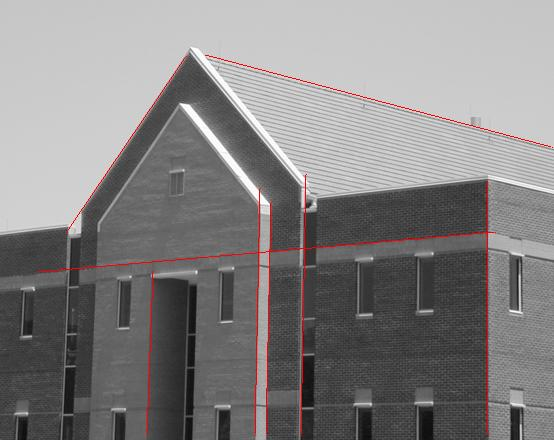展开全文• 基于hough变换直线检测matlab代码，可直接运行，无需配置
•文档
• 首先用canny算子检测图像中的边缘，然后利用hough变换检测边缘中的直线，并显示，matlab实现。
• 利用Hough变换进行直线检测，原始图像经过边缘检测Hough变换

### 这里写自定义目录标题

原创文章作者：无敌三角猫。如若转载，请注明出处：古月居 https://www.guyuehome.com/37662

# 1引言

该程序实现了一些基本的图像处理算法，并将它们组合在一起，构建了一个基于霍夫变换的直线检测器。该程序能够在图像中找到直线段的起始点和结束点。像大多数视觉算法一样，Hough变换使用了一些参数，它们的最优值是具有数据依赖性(即一组参数值在一幅图像上工作得很好，可能对另一幅图像来说不是最好的)。通过在测试图像上运行代码和调优参数，它获得了每个图像的最佳值，从而获得了良好的性能。
● Hough Transform based line detector ：基于Hough变换的直线检测器。
● straight line segments in images：图像中的直线。
● optimal values are data dependent：最优参数具有数据依赖性。

# 2实施

## 2.1卷积

编写一个函数，将图像与给定的卷积滤波器进行变换。

function [img1]=myImageFilter(img0,h)



作为输入，该函数接受存储在矩阵h中的卷积滤波器和灰度图像(img0)。函数的输出应该是与img0相同大小的图像img1，它是由img0和h卷积得到。您可以假设滤波器h沿两个维度都是奇数大小。您将需要处理图像边缘的边界情况。例如，当您在图像的左上角放置卷积掩膜时，大多数滤波器掩膜将位于图像之外。一种可能的解决方案是对图像进行处理，使位于图像边界之外的像素与位于图像内部的最近像素具有相同的强度值。

## 2.2用一个For循环进行卷积

请编写一个只使用一个for循环进行卷积的函数，并将其保存到ec/目录。(如果您在Q3.1中已经这样做了，好的，只需复制一个并保存到EC/。)此外，简单地描述一下你在写作中是如何实现的。插图有助于理解是高度鼓励的。

                           function [img1]=myImageFilterX(img0,h)



为了只使用一个循环，我将2D数组转换成了1D数组，然后使用它们的映射关系。其他代码与2.1完全相同。看.m文件夹ec下。

## 2.3边缘检测

编写一个函数，以查找图像中的边缘强度和方向。在您的写作中包含给定图像之一的功能输出。

function [Im Io Ix Iy]=myEdgeFilter(img,sigma)



该函数将输入灰度图像(img)和σ(标量)。Sigma是高斯平滑核在边缘检测前使用的标准差，函数将输出边缘幅值图像Im，边缘方向图像Io，以及x方向和y方向上的滤波响应Ix和Iy。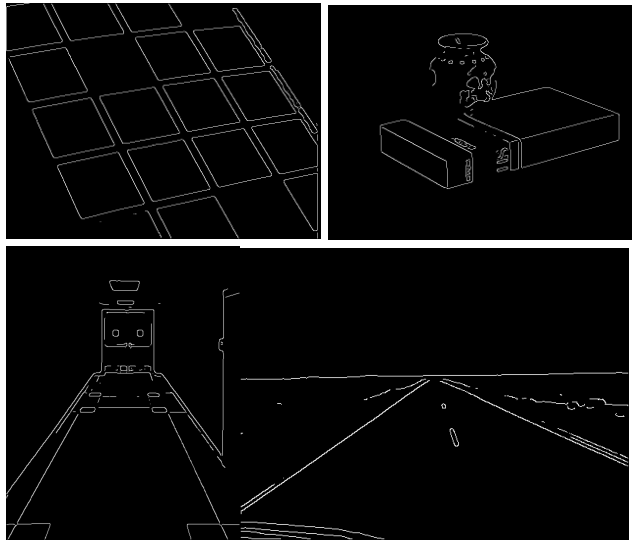## 2.4Hough变换

编写将Hough变换应用于边缘幅值图像的函数。
function [H, rhoScale, thetaScale] = myHoughTransform(Im, threshold, rhoRes, thetaRes)
Im是边缘幅值图像， threshold(标量)是用来忽略像素的边缘强度阈值，该类像素具有低边缘滤波器响应。rhoRes(标量)和ThetaRes(标量)分别是HoughTransform累加器沿ρ轴和θ 轴的分辨率。例如，如果theaRes=5°和，则沿θ轴的线带数为360/5=72。H是Hough变换累加器，包含图像中所有可能的直线“选票”数。rhoscale和thetaScale是myHoughTransform生成Hough变换矩阵H的p和θ 值数组。例如，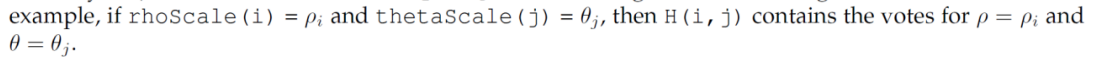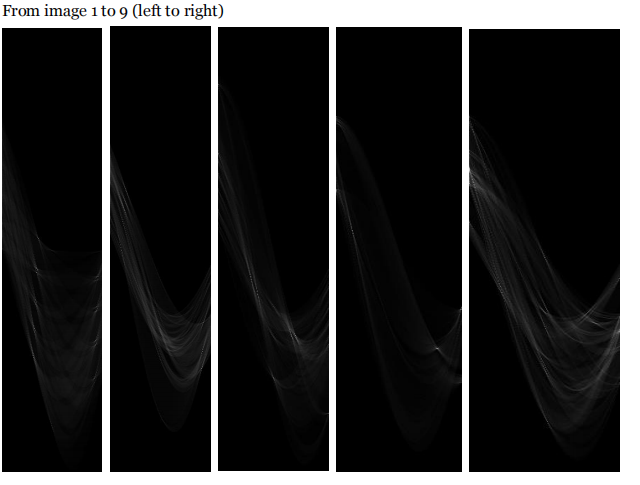## 2.5找直线

function [rhos, thetas] = myHoughLines(H, nLines)



H是Hough变换累加器；RhoRes和taRes是累加器分辨率参数，nLine是返回的行数。Output lineRho和lineTheta都是nline x 1向量，它们分别包含图像中所找到的线条的参数(p和θ)。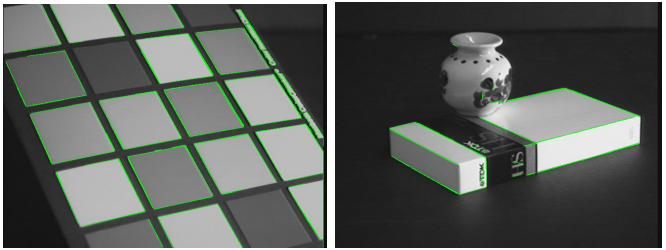(1) Fillgap:如果有不连续的线条需要连接在一起，则将Fillgap设置为相对较高的价值。另一方面，如果有一些不应该在一起的线连接，然后将fillgap设置为一个小值，就像我们在图6中所做的那样。
(2) Minlength:如果图像只有较长的线条，而不是几条较短的线条，则Minlength应该是大的，反之亦然。（3）变化"最小长度": Minlength = 10(左)和Minlength = 30(右)，通过增加线的最小长度可以消除图片中的短噪声线。 根据不同的图片，情况也不同。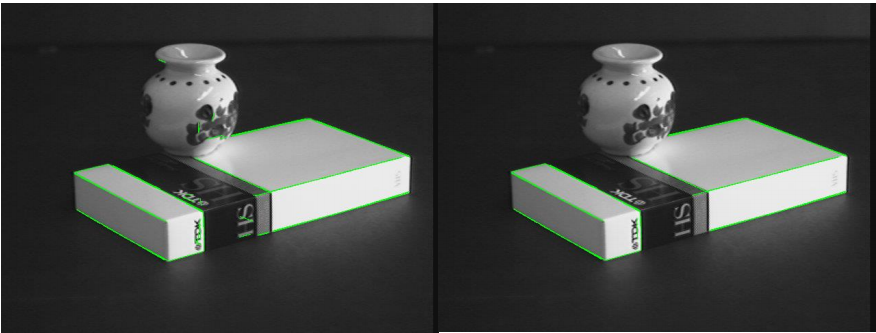# 3讨论

我的代码可以用一个参数对所有的图像，但结果并不理想。
从上图可以看出: (3)阈值:如果边缘图像中图像的线条不清晰，我们应该将阈值设置为 一个相对较低的值来增强它。同样，我们应该设置一个更高的阈值来降低噪音，如果边缘已经足够清晰。
(4) thetaRes和rhoRes:增加这些值可以在Hough投票中获得更好的分辨率图片，在我的代码中rhoRes=pi/180和thetaRes=2工作得最好。
(5) nLine:如果图像中有很多直线需要匹配，那么应该选择一个较大的nLine。 如果有一些线，但大部分是曲线，nLines不应该设置太高。
（6）Fillgap:如果有不连续的线条需要连接在一起，则将Fillgap设置为a 相对较高的价值。另一方面，如果有一些不应该在一起的线连接，然后将fillgap设置为一个小值，就像我们在图6中所做的那样。
（7）Minlength:如果图像只有较长的线条，而不是几条较短的线条，则Minlength应该是大的，反之亦然。
在改变分辨率之前，强制条款部分造成的问题最多。 因为如果分辨率较低，应在附近的霍夫峰仅视为一个峰。所以，有些线找不到。改变分辨率后，问题解决了。但是，对于每个元素在霍夫矩阵中，仍然存在一个问题它的外围可能存在相同的值。我们只能将值设置为0时，有一个值大于它在邻域。所以它可能导致在最后的线拟合过程中有双线。有一种解决方法，就是检查是否有相邻元素中相同的元素只保留其中一个，但它需要一个循环。我的最佳性能参数包括在上面的记录中，还有另一对不同图像的参数。如果对所有的图像只应用一对参数，那将是:
Sigma = 2; threshold = 0.03;
rhoRes = 2; thetaRes = pi/180;
nLines = 50; ‘FillGap’ = 6; ‘MinLength’ = 15；
The effect of single parameter has already been stated above.

## 测试结果

原创文章作者：无敌三角猫。如若转载，请注明出处：古月居 https://www.guyuehome.com/37662

展开全文• 使用霍夫变换检测直线matlab程序，可以求出直线的方程
• Hough变换的原理：将图像从图像空间变换至参数空间，变换公式如下：变换以后，图像...下面使用Matlab实现Hough变换对图像中的直线划痕进行检测。close all;clear all;I = imread('scratch.tif');figure;subplot(1,3,...

Hough变换的原理：

将图像从图像空间变换至参数空间，变换公式如下：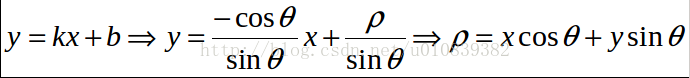变换以后，图像空间与参数空间存在以下关系：

图像空间中的一点在参数空间是一条曲线，而图像空间共线的各点对应于参数空间交于一点的各条曲线。

下面使用Matlab实现Hough变换对图像中的直线划痕进行检测。

close all;

clear all;

figure;

subplot(1,3,1);

imshow(I);

BW = edge(I,'canny');%Canny方法提取图像边界，返回二值图像(边界1,否则0)

[H,T,R] = hough(BW);%计算二值图像的标准霍夫变换，H为霍夫变换矩阵，I,R为计算霍夫变换的角度和半径值

subplot(1,3,2);

imshow(H,[],'XData',T,'YData',R,'InitialMagnification','fit');%hough变换的图像

xlabel('\theta'), ylabel('\rho');

axis on,axis square,hold on;

P = houghpeaks(H,3);%提取3个极值点

x = T(P(:,2));

y = R(P(:,1));

plot(x,y,'s','color','white');%标出极值点

lines=houghlines(BW,T,R,P);%提取线段

subplot(1,3,3);

imshow(I), hold on;

for k = 1:length(lines)

xy = [lines(k).point1; lines(k).point2];

plot(xy(:,1),xy(:,2),'LineWidth',2,'Color','green');%画出线段

plot(xy(1,1),xy(1,2),'x','LineWidth',2,'Color','yellow');%起点

plot(xy(2,1),xy(2,2),'x','LineWidth',2,'Color','red');%终点

end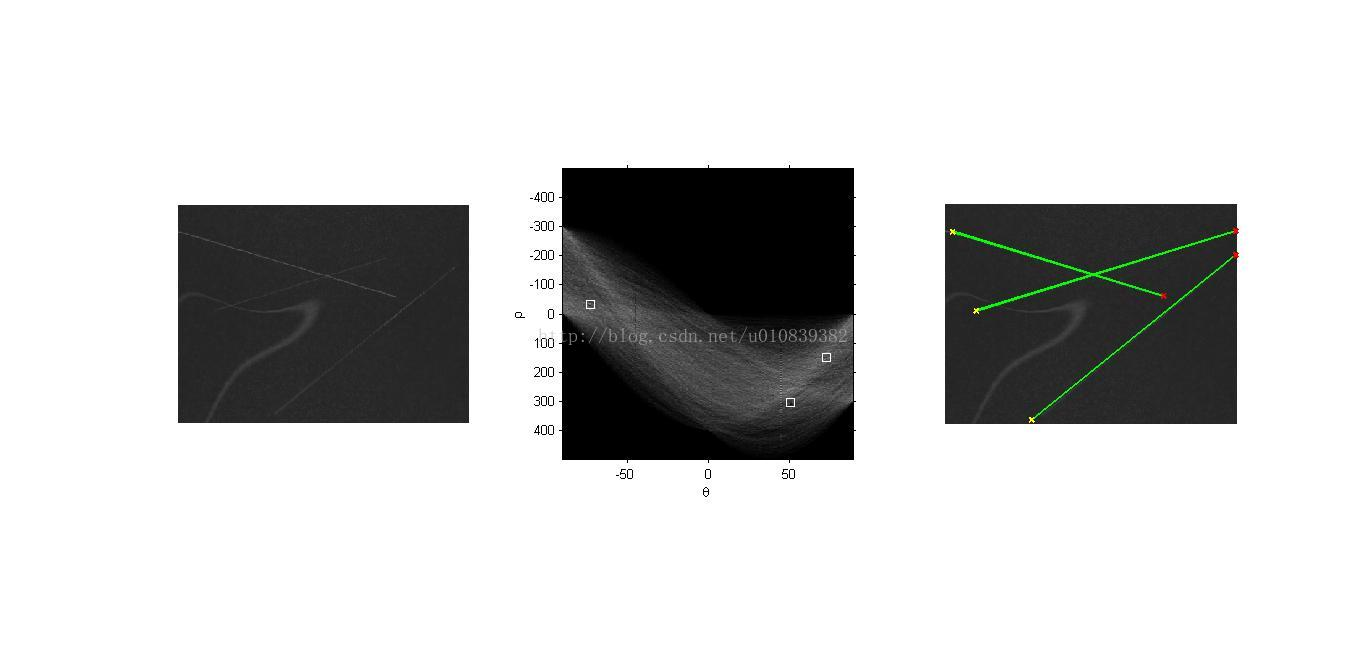Matlab实现Hough变换检测图像中的直线

时间： 06-15

展开全文• hough变换检测直线matlab代码直线提取 该算法基于线支持区域概念（Burns'86），该方法首先检测包含具有相似梯度方向的连接像素的区域，然后推断基于线方向和中心位置的检测区域。 主要区别在于我们使用结构张量来...
• 网上找到的。想了解Hough霍夫曼直线检测效果的，可以下来学习
• 1.Hough变换是一个非常重要的检测间断点边界形状的方法，它通过将图像坐标空间变换到参数空间，来实现直线和曲线的拟合。 2.通过Hough变换，在二值图像中检测直线需要三个步骤  a)利用hough()函数执行hough变换，...
•opencv 计算机视觉 算法
• Hough变换的定义 :Hough变换的基本原理是将影像空间中的曲线变换到参数空间中，通过检测参数空间中的极值点，确定出该曲线的描述参数，从而提取影像中的规则曲线。 知道了定义后了解一下它的性质，你会感叹它...人工智能 图像处理 计算机视觉
• Hough变换直线检测算法的Matlab实现Hough变换的原理简介新的改变功能快捷键合理的创建标题，有助于目录的生成如何改变文本的样式插入链接与图片如何插入一段漂亮的代码片生成一个适合你的列表创建一个表格设定内容...计算机视觉
• 满意答案love8047g2013.05.15采纳率：43%等级：13已帮助：12527人直接运行：RGB = imread('gantrycrane.png');I = rgb2gray(RGB); % convert to intensityBW... % extract edges[H,T,R] = hough(BW,'RhoResolution',...
• 经典的Hough变换是一种常用的检测直线的方法，Hough变换的研究及应用动态：Hough变换于1962年由Paul Hough提出，并在美国作为专利被发表。它所实现的是一种从图像空间到参数空间的映射关系。由于具有一些明显优点和...
• hough变换检测直线->houghpeaks检测峰值->houghlines检测直线 clc,close BW=imread('C:\Users\10493\Desktop\钻石角度测量.bmp');%文件路径 BW= edge(BW,'LOG',0.01)%采用LOG滤波 figu...
• 基于hough变换检测直线，用MATLAB实现 我只是想换积分下个东西。。。
• 霍夫变换于1962年由Paul Hough 首次提出，后于1972年由Richard Duda和Peter Hart推广使用，经典霍夫变换用来检测图像中的直线，后来霍夫变换扩展到任意形状物体的识别，多为圆和椭圆。 霍夫变换运用两个...html5 html css
• Hough变换目的 原始图像中直线检测问题转化为寻找参数空间中的交点个数统计的极值问题。 基本原理 在r-theta参数空间中具有同样（theta，r）的点在一条直线上。 直角坐标系中无法表达垂直直线，极坐标系中，每一条...
• 霍夫变换是一种特征检测(feature extraction)，被广泛应用在图像分析(image analysis)、电脑视觉 (computer vision)以及数位影像处理 (digital image processing)。 霍夫变换是用来辨别找出物件中的特征，例如：线条...
• 使用MATLAB实现Hough变换检测车道线的demo程序
• 智能优化算法、神经网络预测、信号处理、元胞自动机、图像处理、路径规划、无人机等多种领域的Matlab仿真代码
• hough变换检测直线matlab代码楼梯检测 这是一个图像处理项目，旨在识别图像中是否存在楼梯。 捕获了实时图像的数据集，其中包括一些基本障碍物，例如楼梯，纸箱等。以2秒的间隔拍摄图像以测试检测过程的效率。 用法 ...
• matlab_hough变换提取直线 matlab代码，可直接运行 matlab_hough变换提取直线 matlab代码，可直接运行
• 复习久了觉得枯燥，玩玩儿霍夫变换直线检测霍夫变换的基本原理不难，即便是初中生也很容易理解(至少在直线检测上是这样子的)。霍夫变换直线检测的基本原理：(不配图了，自己在白纸上画画，理解更深刻)一步一步来：1...
• 《边缘检测Hough变换实验报告 Matlab - 图文》由会员分享，可在线阅读，更多相关《边缘检测Hough变换实验报告 Matlab - 图文(5页珍藏版)》请在人人文库网上搜索。1、边缘检测Hough变换实验报告 Matlab - 图文...
• ​基于Hough霍夫曼算法实现直线检测matlab代码 2 完整代码 图片自行替换即可 clear;clc;closeall I1=imread('图片\1.bmp'); I=rgb2gray(I1); index=find(I<50); I(index)=0; BW=im2bw(I); [H,T,R]=hough(BW);...算法 开发语言
• 基于Hough 变换直线检测(Matlab实现)  输入图像:  输出图像: 源代码:  (参考Matlab houghlines 例程) clear I = imread('taj1small3.jpg'); I = rgb2gray(I); %I = imrotate(I,33,'...

# hough变换直线检测matlabmatlab 订阅